In :
from matplotlib import pyplot as plt


# Hypergraphs¶

are matematical creatures which prove to be quite useful.

# Generating hypergraphs¶

there are lots of types of hypergraphs, because hypergraphs are pretty general.

Hyperedges are arbitrary sets of nodes, and can therefore contain an arbitrary number of nodes. It is often better study hypergraphs where all hyperedges have the the same number of vertices in hyperedges. (In other words, it is a collection of sets of size k.) So a 2-uniform hypergraph is a graph, a 3-uniform hypergraph is a collection of unordered triples, and so on.

There are also matroids, greedoids and so on... but it gets little complicated.

# Generating uniform hypergraphs¶

My method of generating uniform hypergraphs is pretty straightforward. Generate set of vertices and get some combinations as sets...

# todo¶

• wipe this notebook out and use new libs
• write it with a good plan
In :
import random
import math
import itertools

def generate_hypergraph(n=6, number_of_edges=None, k=3):
vertices = range(1, n + 1)

if number_of_edges is None:
number_of_edges = int(math.sqrt(n))
hyper_edges = random.sample(list(itertools.combinations(vertices, k)), number_of_edges)
return (vertices, hyper_edges)

In :
g = generate_hypergraph(number_of_edges=8)

In :
import networkx as nx

def convert_to_nx_biparty_graph(vertices, hyper_edges):
G = nx.Graph()
for e in hyper_edges:
for n in e:
return G


In :
G = convert_to_nx_biparty_graph(*g)

In :
%matplotlib inline
nx.draw(G)
nx.draw(G,pos=nx.spring_layout(G, iterations=200))
from networkx.algorithms import bipartite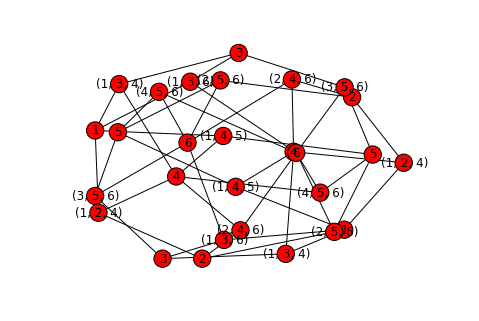In :
def draw_bipartite_graph(G, group_1, group_2):
pos = {x:(0 , float(i % 20) * 2) for i, x in enumerate(group_1)}
pos.update({node: (18.3, 0 + float(i % 20) * 2) for i, node in enumerate(group_2)})

nx.draw(G, pos, node_color='m', node_size=800, with_labels=True, width=1.3, alpha=0.4)

def hipergraph_to_bipartite_parts(G):
group_1 = (node for node in G.nodes() if not isinstance(node, tuple))
group_2 = (node for node in G.nodes() if isinstance(node, tuple))
return group_1, group_2

draw_bipartite_graph(G, *hipergraph_to_bipartite_parts(G))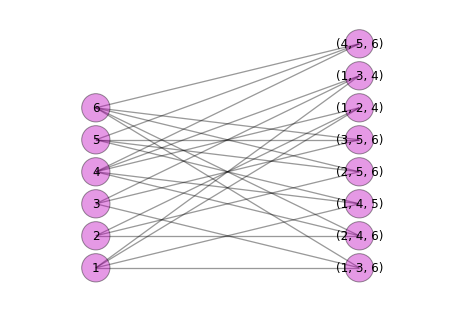In :
hyper_edges = g

In :
hyper_edges

Out:
[(1, 2, 4),
(1, 4, 5),
(4, 5, 6),
(1, 3, 6),
(2, 4, 6),
(1, 3, 4),
(3, 5, 6),
(2, 5, 6)]
In :
from itertools import chain

def convert_to_custom_hyper_G(nodes, edges):
nodes = [node for node in edges if isinstance(node, tuple)]
edges = list(chain(*[[(node_1, node_2) for x in range(len(set(node_1) & set(node_2)))] for node_1, node_2 in itertools.combinations(edges, 2) if set(node_1) & set(node_2)]))
hyper_G = nx.Graph()
return hyper_G

In :
%matplotlib inline
hyper_G = convert_to_custom_hyper_G(*g)
nx.draw(hyper_G, layout=nx.circular_layout(G), node_size=3000, node_color='g', alpha=0.5)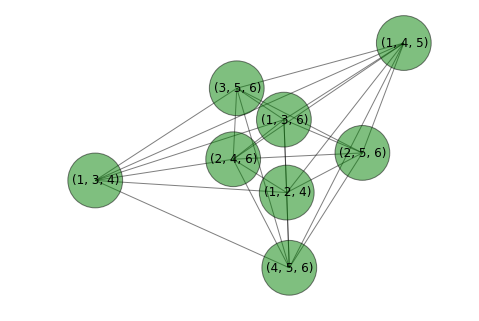In :
nx.degree(hyper_G)

Out:
{(2, 5, 6): 6,
(3, 5, 6): 6,
(1, 2, 4): 6,
(1, 3, 4): 6,
(1, 3, 6): 7,
(2, 4, 6): 7,
(4, 5, 6): 7,
(1, 4, 5): 7}
In :
nx.adjacency_matrix(hyper_G)

Out:
matrix([[ 0.,  1.,  1.,  0.,  1.,  1.,  1.,  1.],
[ 1.,  0.,  0.,  1.,  1.,  1.,  1.,  1.],
[ 1.,  0.,  0.,  1.,  1.,  1.,  1.,  1.],
[ 0.,  1.,  1.,  0.,  1.,  1.,  1.,  1.],
[ 1.,  1.,  1.,  1.,  0.,  1.,  1.,  1.],
[ 1.,  1.,  1.,  1.,  1.,  0.,  1.,  1.],
[ 1.,  1.,  1.,  1.,  1.,  1.,  0.,  1.],
[ 1.,  1.,  1.,  1.,  1.,  1.,  1.,  0.]])
In :
nx.incidence_matrix(hyper_G)

Out:
matrix([[ 1.,  1.,  1.,  1.,  1.,  1.,  0.,  0.,  0.,  0.,  0.,  0.,  0.,
0.,  0.,  0.,  0.,  0.,  0.,  0.,  0.,  0.,  0.,  0.,  0.,  0.],
[ 1.,  0.,  0.,  0.,  0.,  0.,  1.,  1.,  1.,  1.,  1.,  0.,  0.,
0.,  0.,  0.,  0.,  0.,  0.,  0.,  0.,  0.,  0.,  0.,  0.,  0.],
[ 0.,  1.,  0.,  0.,  0.,  0.,  0.,  0.,  0.,  0.,  0.,  1.,  1.,
1.,  1.,  1.,  0.,  0.,  0.,  0.,  0.,  0.,  0.,  0.,  0.,  0.],
[ 0.,  0.,  0.,  0.,  0.,  0.,  1.,  0.,  0.,  0.,  0.,  1.,  0.,
0.,  0.,  0.,  1.,  1.,  1.,  1.,  0.,  0.,  0.,  0.,  0.,  0.],
[ 0.,  0.,  1.,  0.,  0.,  0.,  0.,  1.,  0.,  0.,  0.,  0.,  1.,
0.,  0.,  0.,  1.,  0.,  0.,  0.,  1.,  1.,  1.,  0.,  0.,  0.],
[ 0.,  0.,  0.,  1.,  0.,  0.,  0.,  0.,  1.,  0.,  0.,  0.,  0.,
1.,  0.,  0.,  0.,  1.,  0.,  0.,  1.,  0.,  0.,  1.,  1.,  0.],
[ 0.,  0.,  0.,  0.,  1.,  0.,  0.,  0.,  0.,  1.,  0.,  0.,  0.,
0.,  1.,  0.,  0.,  0.,  1.,  0.,  0.,  1.,  0.,  1.,  0.,  1.],
[ 0.,  0.,  0.,  0.,  0.,  1.,  0.,  0.,  0.,  0.,  1.,  0.,  0.,
0.,  0.,  1.,  0.,  0.,  0.,  1.,  0.,  0.,  1.,  0.,  1.,  1.]])
In :
import numpy as np
from matplotlib import pyplot as plt
from collections import Counter

def show_different_hipergraphs(n=10, k=3, parts=10):
for fraction in np.linspace(0, 1, parts)[1:]:
plt.figure(figsize=(6, 6))
g = generate_hypergraph(n=n, k=k, number_of_edges=int(n * fraction))
G = convert_to_nx_biparty_graph(*g)
draw_bipartite_graph(G, *hipergraph_to_bipartite_parts(G))
hyper_G = convert_to_custom_hyper_G(*g)

p=nx.degree(hyper_G)

plt.figure(figsize=(6, 6))
nx.draw(
hyper_G, node_color=p.values(),
node_size=3000,
cmap=plt.cm.Blues,
alpha=0.6
)


In :
show_different_hipergraphs(10, 3, 4)

---------------------------------------------------------------------------
ValueError                                Traceback (most recent call last)
<ipython-input-21-864842f706f8> in <module>()
----> 1 show_different_hipergraphs(10, 3, 4)

<ipython-input-20-a6a09d85a617> in show_different_hipergraphs(n, k, parts)
18             node_size=3000,
19             cmap=plt.cm.Blues,
---> 20             alpha=0.6
21         )
22

/home/att/Projects/hypergraph/py3/lib/python3.4/site-packages/networkx/drawing/nx_pylab.py in draw(G, pos, ax, hold, **kwds)
124         plt.hold(h)
125     try:
--> 126         draw_networkx(G,pos=pos,ax=ax,**kwds)
127         ax.set_axis_off()
128         plt.draw_if_interactive()

/home/att/Projects/hypergraph/py3/lib/python3.4/site-packages/networkx/drawing/nx_pylab.py in draw_networkx(G, pos, with_labels, **kwds)
257         pos=nx.drawing.spring_layout(G) # default to spring layout
258
--> 259     node_collection=draw_networkx_nodes(G, pos, **kwds)
260     edge_collection=draw_networkx_edges(G, pos, **kwds)
261     if with_labels:

/home/att/Projects/hypergraph/py3/lib/python3.4/site-packages/networkx/drawing/nx_pylab.py in draw_networkx_nodes(G, pos, nodelist, node_size, node_color, node_shape, alpha, cmap, vmin, vmax, ax, linewidths, label, **kwds)
379                                alpha=alpha,
380                                linewidths=linewidths,
--> 381                                label=label)
382
383     node_collection.set_zorder(2)

/usr/lib/python3/dist-packages/matplotlib/axes.py in scatter(self, x, y, s, c, marker, cmap, norm, vmin, vmax, alpha, linewidths, verts, **kwargs)
6278                 colors = None  # use cmap, norm after collection is created
6279             else:
-> 6280                 colors = mcolors.colorConverter.to_rgba_array(c, alpha)
6281
6282         faceted = kwargs.pop('faceted', None)

/usr/lib/python3/dist-packages/matplotlib/colors.py in to_rgba_array(self, c, alpha)
379         except TypeError:
380             raise ValueError(
--> 381                 "Cannot convert argument type %s to rgba array" % type(c))
382         try:
383             if nc == 0 or c.lower() == 'none':

ValueError: Cannot convert argument type <class 'numpy.ndarray'> to rgba array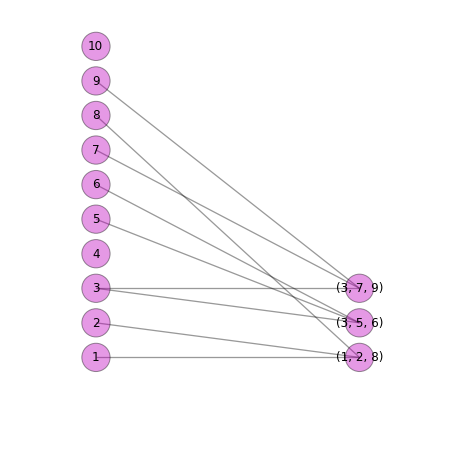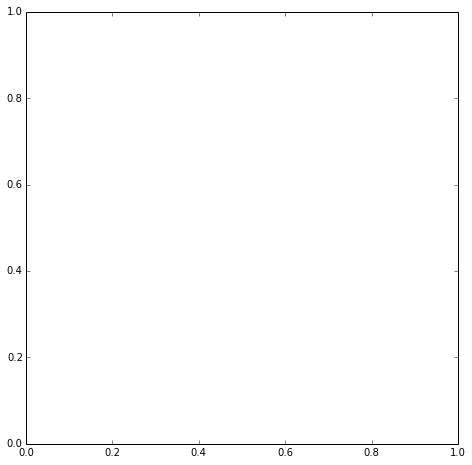In :
A = np.array([[1, 2], [2, 0]])
min(A.sum(axis=0))

Out:
2
In :
def create_balanced_markov_matrix(edges):
a_matrix = np.zeros([len(edges), len(edges)]) * 10
for i, edge_1 in enumerate(edges):
for j, edge_2 in enumerate(edges):
a_matrix[i][j] = len(set(edge_1) & set(edge_2))
a_matrix[j][i] = a_matrix[i][j]
sums = a_matrix.sum(axis=0)
min_sum = min(sums)
for i, col_sum in enumerate(sums):
a_matrix[i][i] -= col_sum - min_sum
a_matrix *= 1.0 /min_sum
return a_matrix

def create_markov_matrix(edges):
a_matrix = np.eye(len(edges))

for i, edge_1 in enumerate(edges):
for j, edge_2 in enumerate(edges):
a_matrix[i][j] = len(set(edge_1) & set(edge_2))
a_matrix[j][i] = a_matrix[i][j]

for i, edge_1 in enumerate(edges):
a_matrix[i] = a_matrix[i] / sum(a_matrix[i]) * 1.1

return a_matrix

In :
create_markov_matrix(g)

Out:
array([[ 0.275     ,  0.18333333,  0.09166667,  0.09166667,  0.18333333,
0.18333333,  0.        ,  0.09166667],
[ 0.16923077,  0.25384615,  0.16923077,  0.08461538,  0.08461538,
0.16923077,  0.08461538,  0.08461538],
[ 0.07857143,  0.15714286,  0.23571429,  0.07857143,  0.15714286,
0.07857143,  0.15714286,  0.15714286],
[ 0.09166667,  0.09166667,  0.09166667,  0.275     ,  0.09166667,
0.18333333,  0.18333333,  0.09166667],
[ 0.16923077,  0.08461538,  0.16923077,  0.08461538,  0.25384615,
0.08461538,  0.08461538,  0.16923077],
[ 0.18333333,  0.18333333,  0.09166667,  0.18333333,  0.09166667,
0.275     ,  0.09166667,  0.        ],
[ 0.        ,  0.09166667,  0.18333333,  0.18333333,  0.09166667,
0.09166667,  0.275     ,  0.18333333],
[ 0.09166667,  0.09166667,  0.18333333,  0.09166667,  0.18333333,
0.        ,  0.18333333,  0.275     ]])
In :
import numpy as np
from numpy.random import random_sample

def weighted_values(values, probabilities, size):
bins[-1] = max(1, bins[-1])
index = np.digitize(random_sample(size), bins)
return values[index]

def step_diffusion(current_state, markov_matrix):
values_size = len(current_state)
values = range(values_size)
probs = markov_matrix.dot(current_state)
next_state = np.zeros(values_size)
next_state[weighted_values(values, probs, 1)] = 1

return next_state

In :


In :
markov_matrix = create_markov_matrix(g)
current_state = np.zeros(markov_matrix.shape)
current_state = 1
print(current_state)
print(markov_matrix)

[ 1.  0.  0.  0.  0.  0.  0.  0.]
[[ 0.275       0.18333333  0.09166667  0.09166667  0.18333333  0.18333333
0.          0.09166667]
[ 0.16923077  0.25384615  0.16923077  0.08461538  0.08461538  0.16923077
0.08461538  0.08461538]
[ 0.07857143  0.15714286  0.23571429  0.07857143  0.15714286  0.07857143
0.15714286  0.15714286]
[ 0.09166667  0.09166667  0.09166667  0.275       0.09166667  0.18333333
0.18333333  0.09166667]
[ 0.16923077  0.08461538  0.16923077  0.08461538  0.25384615  0.08461538
0.08461538  0.16923077]
[ 0.18333333  0.18333333  0.09166667  0.18333333  0.09166667  0.275
0.09166667  0.        ]
[ 0.          0.09166667  0.18333333  0.18333333  0.09166667  0.09166667
0.275       0.18333333]
[ 0.09166667  0.09166667  0.18333333  0.09166667  0.18333333  0.
0.18333333  0.275     ]]

In :
current_state = 1

from collections import Counter

c = Counter()
states = []
t_max = 30
for _ in range(t_max):
current_state = step_diffusion(current_state, markov_matrix)
visited_hyperedge = current_state.tolist().index(1)
c[visited_hyperedge] += 1
states.append(visited_hyperedge)

In :
c.most_common()

Out:
[(3, 7), (4, 6), (5, 5), (0, 4), (6, 4), (7, 2), (1, 1), (2, 1)]
In :
y, x = c.keys(), c.values()
print(list(x), y)

[4, 1, 1, 7, 6, 5, 4, 2] dict_keys([0, 1, 2, 3, 4, 5, 6, 7])

In :
from matplotlib import pyplot as plt

In :
plt.hist(states, normed=True)

Out:
(array([ 0.19047619,  0.04761905,  0.04761905,  0.        ,  0.33333333,
0.28571429,  0.        ,  0.23809524,  0.19047619,  0.0952381 ]),
array([ 0. ,  0.7,  1.4,  2.1,  2.8,  3.5,  4.2,  4.9,  5.6,  6.3,  7. ]),
<a list of 10 Patch objects>)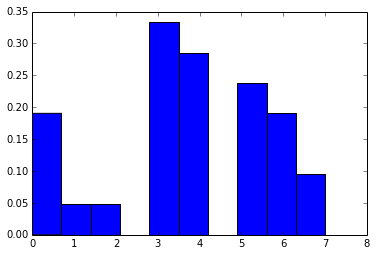In :
g

Out:
(1, 3, 6)
In :
n = 20
k = 3
fraction = 1.0 / 2
g = generate_hypergraph(n=n, k=k, number_of_edges=int(n * fraction))
G = convert_to_nx_biparty_graph(*g)
draw_bipartite_graph(G, *hipergraph_to_bipartite_parts(G))
hyper_G = convert_to_custom_hyper_G(*g)

print("Hyperg")
print(hyper_G)
p=nx.degree(hyper_G)

plt.figure(figsize=(6, 6))

markov_matrix = create_markov_matrix(g)

Hyperg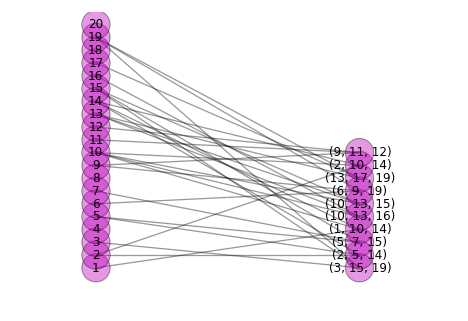<matplotlib.figure.Figure at 0x7f07a48b7a58>
In :
print markov_matrix
print g

  File "<ipython-input-34-880cfd421d4f>", line 1
print markov_matrix
^
SyntaxError: invalid syntax

In :
from collections import Counter
current_state = np.zeros(markov_matrix.shape)
t_max = 100000
current_state = 1
def simulate(current_state, markov_matrix, t_max):
c = Counter()
states = []
for _ in xrange(t_max):
current_state = step_diffusion(current_state, markov_matrix)
visited_hyperedge = current_state.tolist().index(1)
c[visited_hyperedge] += 1
states.append(visited_hyperedge)
return c.most_common(), states

most_common, states = simulate(current_state, markov_matrix, t_max)

print markov_matrix
print most_common

  File "<ipython-input-35-83ff2fbefed3>", line 17
print markov_matrix
^
SyntaxError: invalid syntax

In :
plt.hist(states, alpha=.5);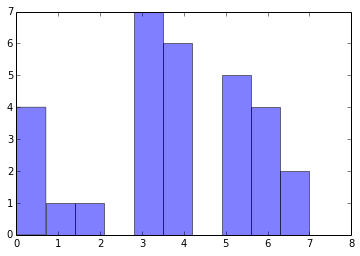In :
def count_nodes(nodes, edges, occurences):
c = Counter()
for index, count in occurences:
for edge in edges[index]:
c[edge] += count
for node in nodes:
if node not in c:
c[node] = 0
return c

In :
most_common_nodes = count_nodes(g, g, most_common)

plt.plot(
most_common_nodes.keys(),
most_common_nodes.values(),
'm:o',
alpha=0.7,
linewidth=5
)

---------------------------------------------------------------------------
NameError                                 Traceback (most recent call last)
<ipython-input-39-3cc5ef1d1ace> in <module>()
----> 1 most_common_nodes = count_nodes(g, g, most_common)
2
3 plt.plot(
4     most_common_nodes.keys(),
5     most_common_nodes.values(),

NameError: name 'most_common' is not defined
In :


In :